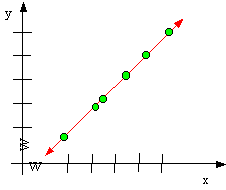### CFA Practice Question

There are 208 practice questions for this study session.

### CFA Practice Question

For a data collection of x and y scores, if a straight line with a positive slope fits the data perfectly thenA. the y-intercept is (0, 1)
B. the correlation coefficient is +1
C. the slope value is 0.5
Explanation: A correlation coefficient of +1 indicates that the x-y data pairs are such that a straight line with a positive slope fits the data perfectly.